The Nature of Mathematical Modeling - Rachelle Villalon

Function Fitting

 I must admit, I have never seen nor used an SVD (Singular Value Decomposition) to fit a line to a data set before. In order to overcome this unfamiliarity, I began by computing an SVD by hand to a small matrix and learning along the way (from Neil's notes) that a rectangular matrix can be broken down into a product of three matrices: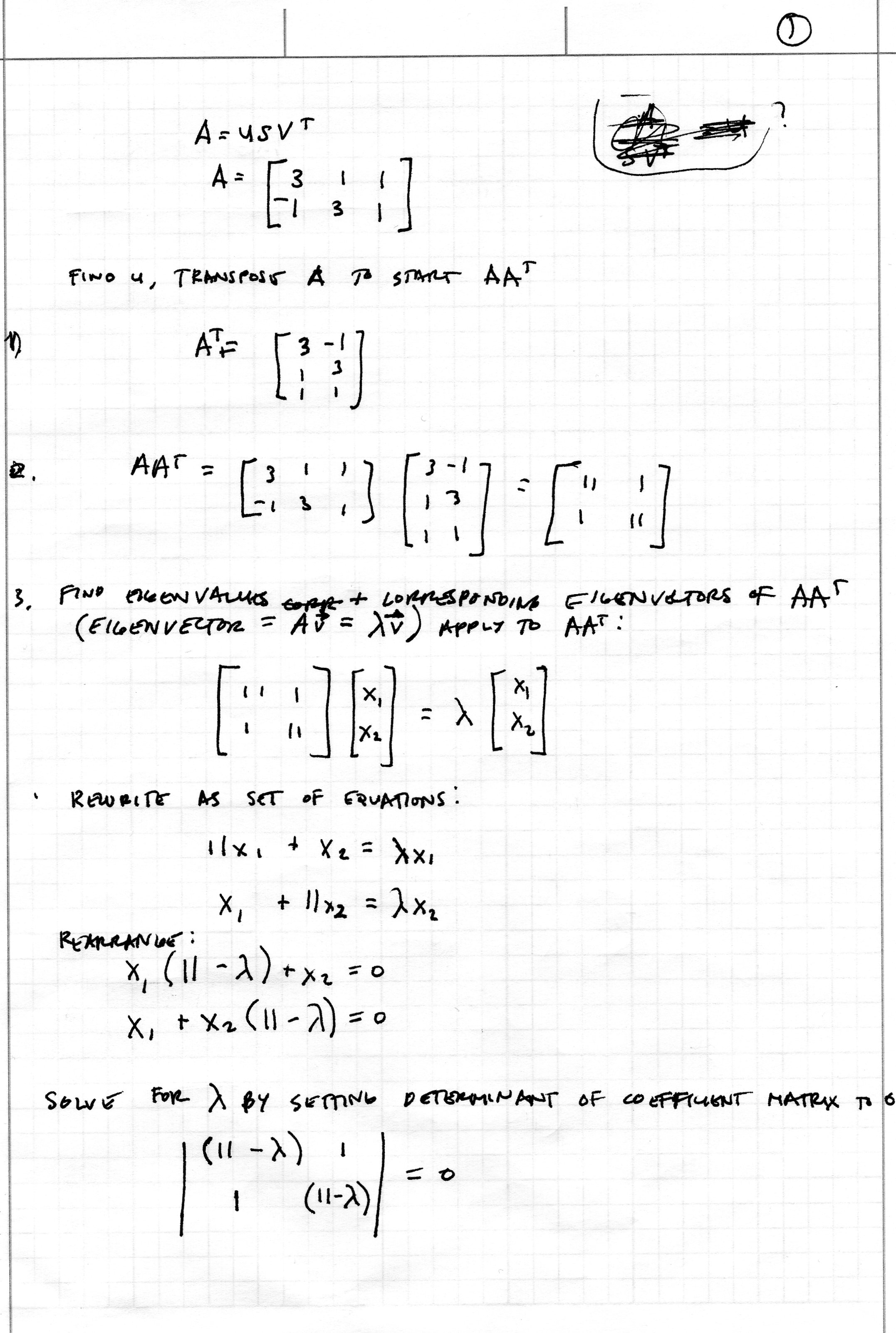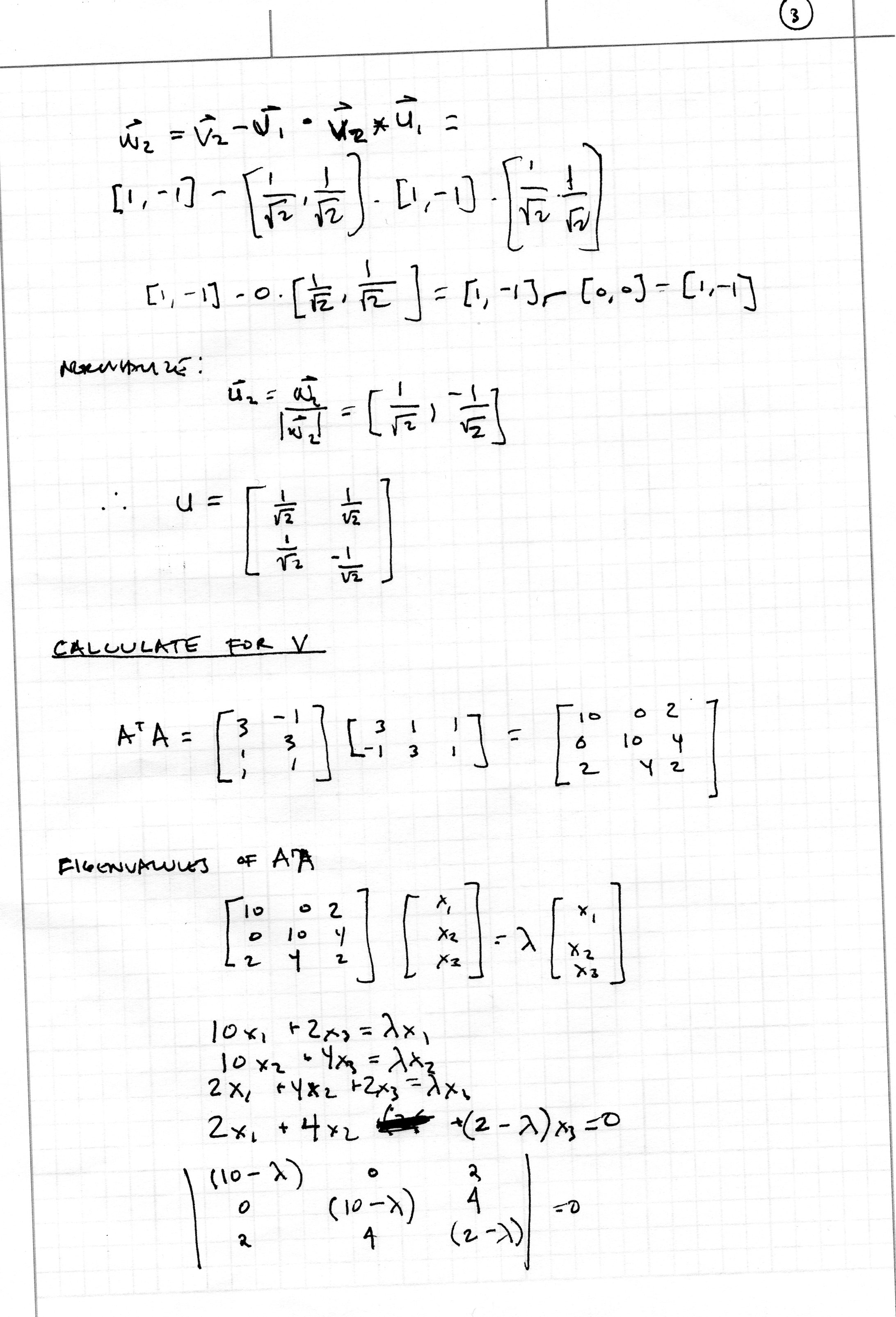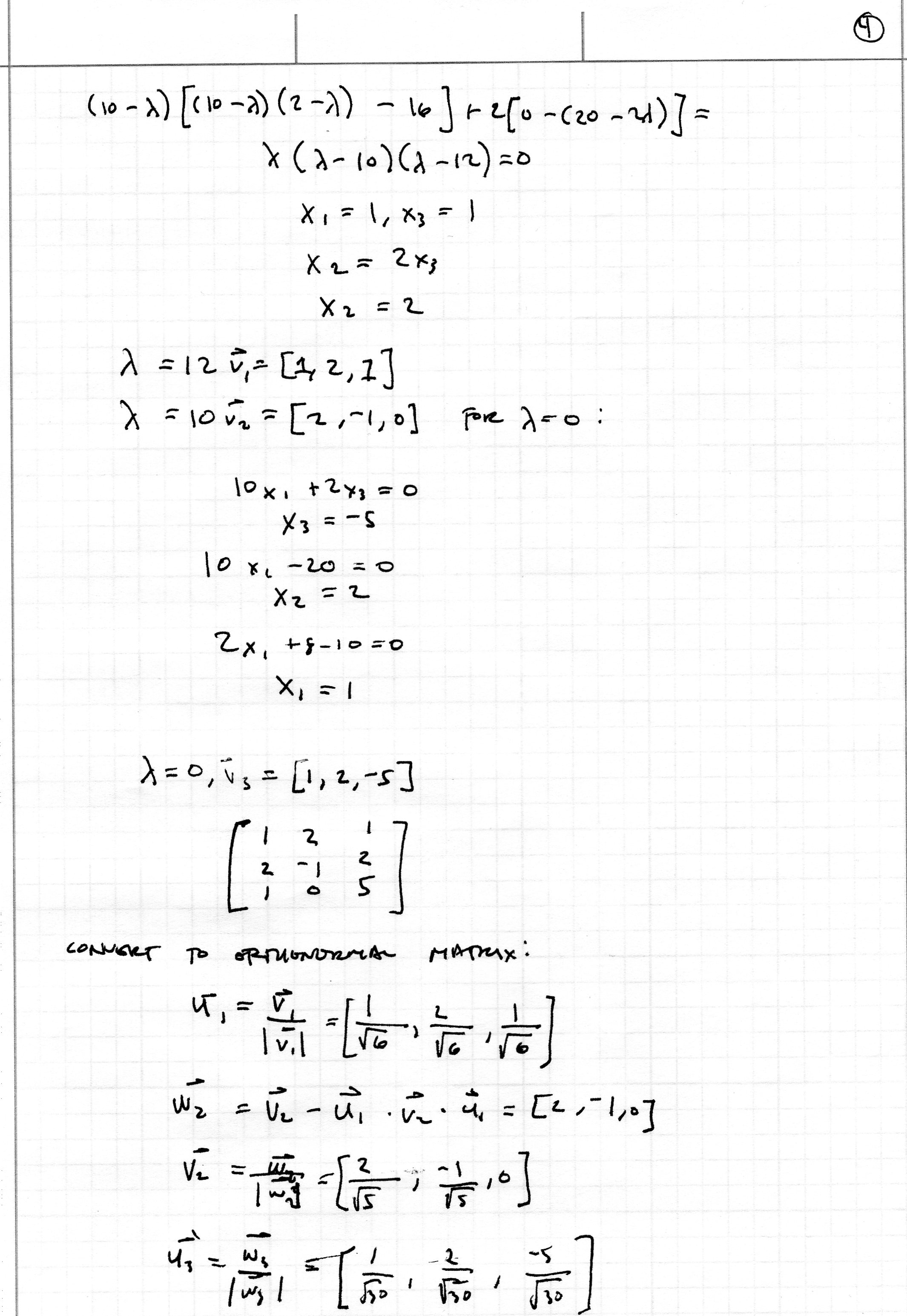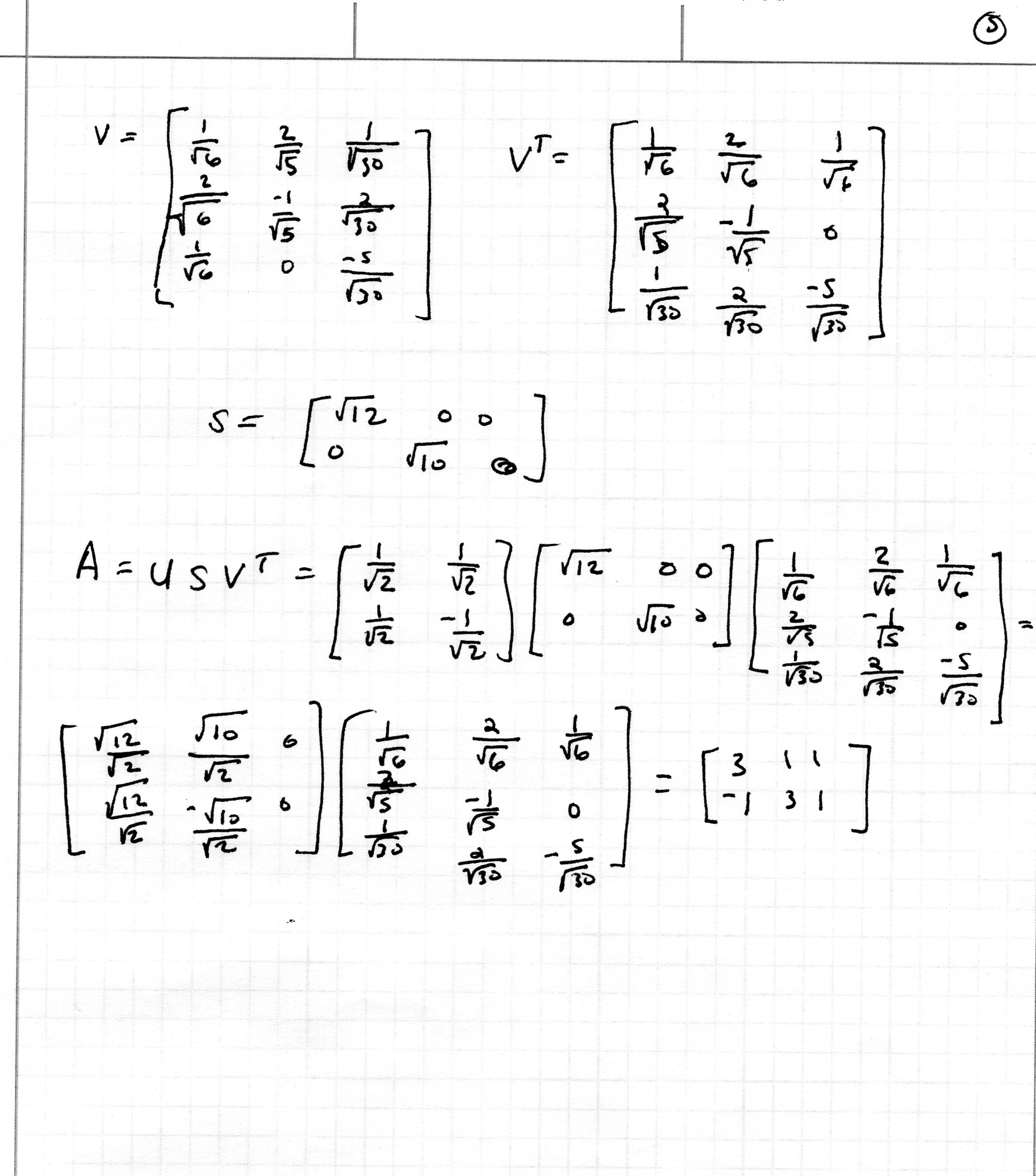Translating the concept into Python for generating 100 points between 0 and 1, letting y = 2 + 3x + g where "g" is a gaussian random variable, yields:Attempts at knowing a data set drawn from a Gaussian distribution to find an optimal estimator of the mean (with the help of fellow NMM classmates):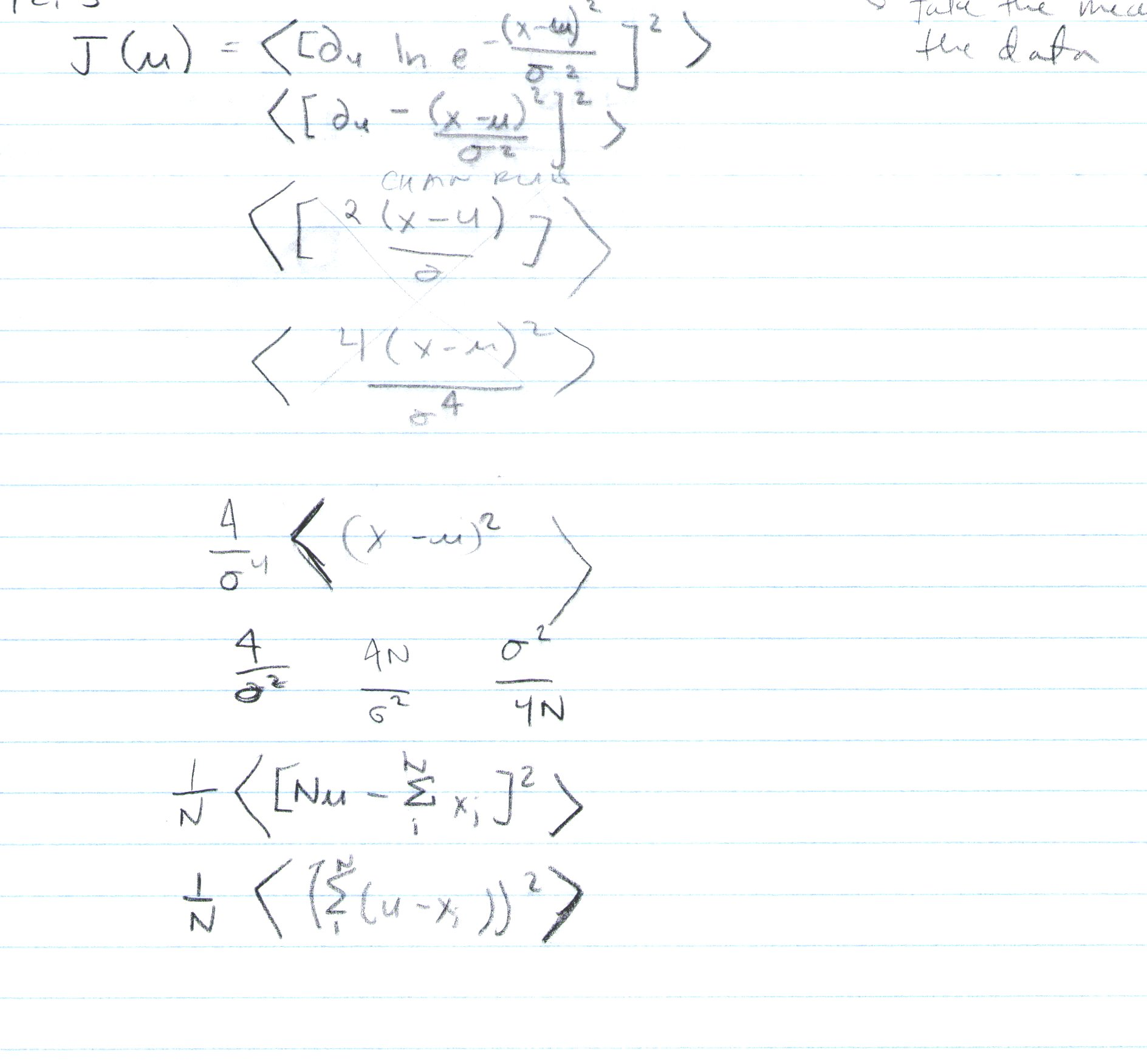(Some) References Online .textContainer { font-family: "HelveticaNeue-Light", "Helvetica Neue Light", "Helvetica Neue", Helvetica, Arial, "Lucida Grande", sans-serif; font-weight: 300; font-size: 15px; }